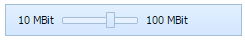Check documentation for the latest version of dhtmlxSuite Slider Control DHTMLX Docs

# Slider Control

an item having a slider in it that allows the user to change item's value within the available limits (the user can drag the slider's thumb in one of two directions):The addSlider method should be used to create a new Slider:

``myToolbar.addSlider(id, pos, len, valueMin, valueMax, valueNow, textMin, textMax, tip);``

As for input parameters the user should set, they are as follows:

• id - id of the new slider item;
• pos - position of the new slider;
• len - slider's length (or width/height, it is set in pixels);
• valueMin - minimum possible value;
• valueMax - maximum possible value;
• valueNow - the value slider is set to for the moment it is created;
• textMin - the text displayed to the left of the slider (in case of horizontal slider display) or below the slider (in case of vertical slider display);
• textMax - the text displayed to the right of the slider (in case of horizontal slider display) or above the slider (in case of vertical slider display);
• tip - this parameter sets the tooltip text.

If you want Slider to be created with a text-free tooltip, it's necessary to set an empty string as a value of the tip parameter.

Related sample:  Slider creation

## Working with Slider

The following methods that are available for the Button item, can be applied to the Slider as well:

### Showing/Hiding Slider

• myToolbar.showItem(id);
• myToolbar.hideItem(id);
• myToolbar.isVisible(id);

Related sample:  Slider manipulation

### Enabling/Disabling Slider

• myToolbar.enableItem(id);
• myToolbar.disableItem(id);
• myToolbar.isEnabled(id).

Related sample:  Slider state

All the parameters of the above mentioned methods are the same to those described in Button Settings Manipulations section.

## Setting Slider's Tooltip Template

Tooltip template is applied only to Slider. The tooltip template can be represented in three ways:

• As a static text, for example, "Hello";
• As a changeable text when the user moves the slider thumb. "%v" part should be added to the tooltip template to make the template changeable;
• Using both above mentioned ways together.

The setItemToolTipTemplate method is used to set the tooltip template:

``myToolbar.setItemToolTipTemplate(id, template);``

This method takes the following parameters:

• id - item id;
• template - tooltip template text itself.

For example, if the user wants his tooltip to show the text like this: "Current Value is:" plus the changeable text showing slider's values when the thumb is moved, the code must be the following:

``myToolbar.setItemToolTipTemplate("slider","Current Value is:+%v");``

The following method can return the current slider's tooltip template:

``var template = myToolbar.getItemToolTipTemplate(id); // returns current slider's tooltip text``

Related sample:  Slider tooltip template

## Setting Slider's Value

The user can set the Slider to a certain value within the range of available values with the help of the following method:

``myToolbar.setValue(id,value,callEvent);``

The parameters are as follows:

• id - id of the item;
• value - the new value;
• callEvent - true/false, if set to true, calls onValueChange event.

Note: If the user has indicated the value that is not within the range of available values, the slider's thumb will be set either to the minimum or to the maximum value.

Slider's current value can be easily got by the following method:

``var value = myToolbar.getValue(id); // returns slider's current value``

Related sample:  Slider value

## Setting Slider's Minimum Value

There is a method to set slider's minimum value:

``myToolbar.setMinValue(id, value, label);``

The following parameters should be passed to this method:

• id - item id;
• value - minimum value;
• label - text displayed to the left of the slider (in case of horizontal slider display) or below the slider (in case of vertical slider display).

Note: if the user wants the slider's minimum value to be created text-free, it's necessary to write an empty string as label parameter. For example:

``myToolbar.setMinValue("slider", 25, "");``

Slider's current minimum value can be got using getMinValue method:

``var data = myToolbar.getMinValue(id); // returns slider's current minimum value``

This method returns the following Array(value, label).

Related sample:  Slider value

## Setting Slider's Maximum Value

There is a method to set slider's maximum value:

``myToolbar.setMaxValue(id,value,label);``

The following parameters should be passed to this method:

• id - item id;
• value - maximum value;;
• label - text displayed to the right of the slider (in case of horizontal slider display) or above the slider (in case of vertical slider display).

Note: if the user wants the slider's maximum value to be created text-free, it's necessary to write an empty string as label parameter. For example:

``myToolbar.setMaxValue("slider", 25, "");``

Slider's current maximum value can be got using getMaxValue method:

``var data = myToolbar.getMaxValue(id); // returns current maximum value``

This method returns the following Array(value, label).

Related sample:  Slider value